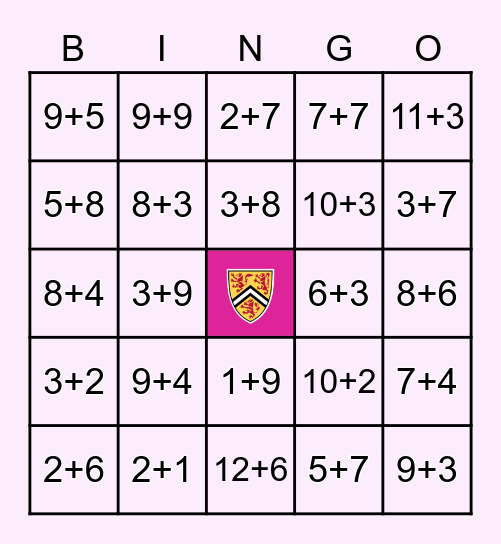# Faculty of Math BingoThis bingo card has a free space and 115 words: 1+1, 2+2, 3+3, 4+4, 5+5, 6+6, 7+7, 8+8, 9+9, 1+2, 1+3, 1+4, 1+5, 1+6, 1+7, 1+8, 1+9, 2+3, 2+4, 2+5, 2+6, 2+7, 2+8, 2+9, 3+1, 3+2, 2+1, 3+4, 3+5, 3+6, 3+7, 3+8, 3+9, 4+1, 4+2, 4+3, 4+5, 4+6, 4+7, 4+8, 4+9, 5+1, 5+2, 5+3, 5+4, 5+6, 5+7, 5+8, 5+9, 6+1, 6+2, 6+3, 6+4, 6+5, 6+7, 6+8, 6+9, 7+1, 7+2, 7+3, 7+4, 7+5, 7+6, 7+8, 7+9, 8+1, 8+2, 8+3, 8+4, 8+5, 8+6, 8+7, 8+9, 9+1, 9+2, 9+3, 9+4, 9+5, 9+6, 9+7, 9+8, 9+9, 10+1, 10+2, 10+3, 10+4, 10+5, 10+6, 10+7, 10+8, 10+9, 10+10, 11+1, 11+2, 11+3, 11+4, 11+5, 11+6, 11+7, 11+8, 11+9, 11+10, 11+11, 12+1, 12+2, 12+3, 12+4, 12+5, 12+6, 12+7, 12+8, 12+9, 12+10, 12+11 and 12+12.

⚠ This card has duplicate items: 9+9 (2)

## Play Online